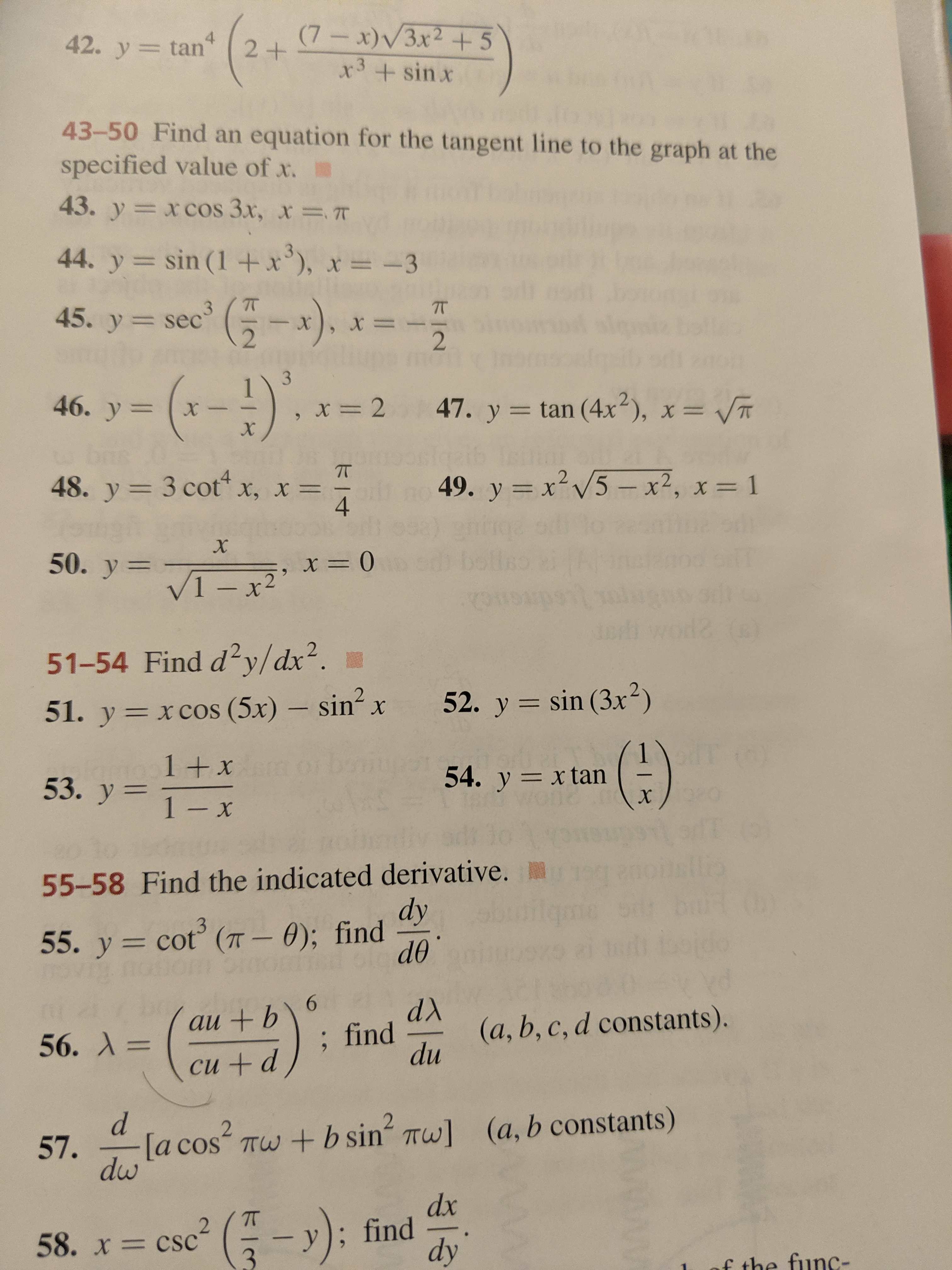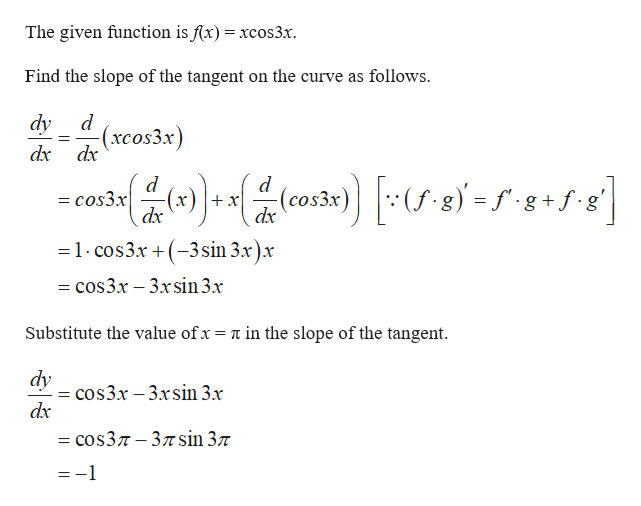# 42. y tanx)/3x2+5x3 + sin x4243-50 Find an equation for the tangent line to the graph at thespecified value of x.43. y= xcos 3x, x7T44. y sin (1 +x), x= -345. y sec x), x==2--)346. y=247. y tan (4x), x = VTX48. y 3 cot x, x4249. y x5- x2, x= 1X50. y 1-2t 051-54 Find dy/dx252. y sin (3x-)51. y xcos (5x) sin xX(G)154. y xtan1 +x53. y 1 Xx55-58 Find the indicated derivative.dy55. y cot (T- 0); finddo6au +b(a, b, c, d constants).du; find56. A=си + d2(a, b constants)[a cos Tw+b sin Tw]57.dwdx- ese -):y; finddy2= CSC358. xf the func-

Question
1 views

#43help_outlineImage Transcriptionclose42. y tan x)/3x2+5 x3 + sin x 4 2 43-50 Find an equation for the tangent line to the graph at the specified value of x. 43. y= xcos 3x, x 7T 44. y sin (1 +x), x= -3 45. y sec x), x == 2 --) 3 46. y =2 47. y tan (4x), x = VT X 48. y 3 cot x, x 4 2 49. y x5- x2, x= 1 X 50. y 1-2t 0 51-54 Find dy/dx2 52. y sin (3x-) 51. y xcos (5x) sin x X (G) 1 54. y xtan 1 +x 53. y 1 X x 55-58 Find the indicated derivative. dy 55. y cot (T- 0); find do 6 au +b (a, b, c, d constants). du ; find 56. A= си + d 2 (a, b constants) [a cos Tw+b sin Tw] 57. dw dx - ese -): y; find dy 2 = CSC 3 58. x f the func- fullscreen
check_circle

Step 1help_outlineImage TranscriptioncloseThe given function is f(x) xcos3x. Find the slope of the tangent on the curve as follows dy (xcos3x) dx dx -(c03) )=f-g+fe| d d = cos3x dx g + dx =1 cos3.x 3sin 3x).x =cos3.x -3xsin 3.x Substitute the value ofx = n in the slope of the tangent aycos3x 3xsin 3x dx — cos3л - 3лsin 3л = -1 fullscreen

### Want to see the full answer?

See Solution

#### Want to see this answer and more?

Solutions are written by subject experts who are available 24/7. Questions are typically answered within 1 hour.*

See Solution
*Response times may vary by subject and question.
Tagged in

### Other